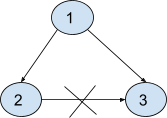# Redundant Connection - II

Posted: 27 Mar, 2021
Difficulty: Moderate

## PROBLEM STATEMENT

#### Note:

``````1. A rooted tree is a directed graph such that, there is exactly one node (the root) for which all other nodes are descendants of this node, plus every node has exactly one parent, except for the root node which has no parents.
2. If there are multiple answers, return the answer that occurs last in the given 2D-array. The answer edge [u, v] should be in the same format, with u < v.
``````
##### Example:
``````Let’s say we have 3 edges that are {1 , 2} , {1 , 3} and {2 , 3}. So the resulting graph from these edges will be :
````````````If we remove the edge {2, 3} then the resulting graph will be a tree
with 'N' nodes.
``````
##### Input format:
``````The very first line of input contains an integer ‘T’ denoting the number of test cases.

The first line of every test case contains an integer ‘N’ denoting the number of nodes of the graph.

The second line of every test case contains a 2D array of 'N' X 2 size which contains 'N' edges of the graph.
``````
##### Output format:
``````For each test case, return that single edge that if removed, the resulting graph will be a tree of 'N' nodes.

Output for each test case is printed on a separate line.
``````
##### Note:
``````1. You do not need to print anything, it has already been taken care of. Just return an edge that can be removed so that the resulting graph is a tree of N nodes.
``````
##### Constraints:
``````1 <= T <= 10
3 <= N <= 1000
1 <= 'ARR[i]' <= N

Where ‘ARR[i]’ represents the edges of the graph.

Time Limit: 1 sec
``````Approach 1

In this approach, we have two cases to handle. The first one is that if a node has two parents then there are two answers and then we have to remove one edge from these two selected answers such that there is no cycle. The other case is that each node has one parent but the graph contains a cycle. So simply remove the edge that causes the cycle to form. Only ‘ANS1’ or ‘ANS2’ can be part of the cycle(if there is a cycle). So, if ‘ANS1’ is empty and cycle found (no 2 parent case, each node has 1 parent), return that edge since it's the last edge found which is part of the cycle. If ‘ANS1’ makes a cycle, return that. Otherwise, return ‘ANS2’ if it makes a cycle. If no cycle is found, then we can simply return ‘ANS2’ as we need to return the last edge in the edges list.

Refer to this doc for disjoint set union https://cp-algorithms.com/data_structures/disjoint_set_union.html

The steps are as follows:

• First, create a struct ‘DSU’ to perform disjoint-set union operations. In the struct define the find operation and union operation.
• We have to traverse over the edges twice. First to check for the nodes that have two parents and next traversal is for cycle detection.
• Create a map ‘PARENT’ to store the parent of a given node in order to identify if a node has two parents.
• Also, create two arrays ‘ANS1’ and ‘ANS2’ to store the edges when a node has two parents.
• Traverse over the given array of edges ‘ARR’ and for every edge do the following:
• Find the source and the target from each edge.
• Check that a node has already a parent or not. This is done as follows:
• if('PARENT'[TARGEt] > 0) then there two edges at that node i.e 'ANS1' = {'PARENT'[TARGET] , ‘TARGET’} and ‘ANS2’ = {'SOURCE', ‘TARGET’}.
• If not then simply do PARENT'[‘TARGET’] = 'SOURCE'.
• In the second traversal, we check which edge out of those two causes cycle.
• If 'ANS2' = current Edge ‘ARR[i]’ then simply continue to the next edge in iteration because we have to return the last edge if there are multiple answers.
• If not find 'SOURCE' and target and call union operation of structure ‘DSU’ and if it returns false that means there is a cycle then:
• If ‘ANS1’ is empty then return the current edge ‘ARR[i]’.
• Otherwise, return ‘ANS1’.
• If the second traversal does not return an edge then return ‘ANS2’ as the answer.